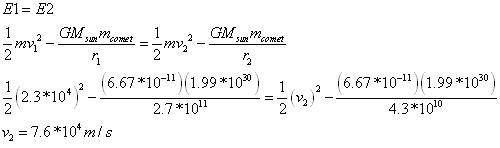## Homework Statement

Comets travel around the sun in elliptical orbits with large eccentricities. If a comet has speed 2.3×10^4 m/s when at a distance of 2.7×10^11 m from the center of the sun, what is its speed when at a distance of 4.3×10^10 m.

## Homework Equations

I tried using v = ((G*M) / r) ^ 0.5, but I'm not sure if that will work with this problem.

## The Attempt at a Solution

* I tried using conservation of angular momentum (per a tutor's advice), but didn't get the right answer
* I tried using Kepler's 2nd Law (area sweep) because the area that is swept out is the same all around the circle, but again no luck
* I tried using conservation of energy
* I used the equation that I listed above and solved for the mass of the comet by plugging in the 1st velocity and radius. Then I used the mass I came up with, along with the 2nd radius and solved for the velocity (v = 5.8*10^4 m/s). I inputted this answer into my online homework site, and it said that I was close, but not right.

dynamicsolo
Homework Helper
I tried using v = ((G*M) / r) ^ 0.5, but I'm not sure if that will work with this problem.

This is the circular velocity for an orbit of radius r, so this pretty definitely won't work for the elliptical orbit of a comet.

I tried using conservation of energy...

This is the only approach that will help you. How did you use it? What is the energy that is conserved as the comet travels on its orbit?

This is what I did with the energy methods:Last edited:
dynamicsolo
Homework Helper
The calculation appears to be all right for the numbers given. The speed of the comet increases by a modest factor of about three for having gotten about six times closer to the Sun.

But I can't help wondering if the distances are given correctly. The comet is said to start at a distance of 2.7·10^11 m , which is only about 1.8 AU, with a speed of 23 km/sec. (The final distance of 4.3·10^10 m is about 0.29 AU, which is credible for a perihelion distance.) That initial speed seems suspiciously low for that distance on a cometary orbit. Might the starting distance be more like 18 AU?

D H
Staff Emeritus